# High School Chemistry : Stoichiometry

## Example Questions

← Previous 1 3

### Example Question #1 : Stoichiometry

Consider the following reaction: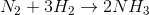How much ammonia will be created if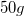of nitrogen gas is used in the reaction?

Possible Answers: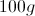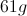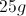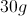Correct answer:Explanation:

Since we are given the mass of nitrogen gas that will be used, we will need to convert the amount into moles. We can then compare the molar ratios in the balanced reaction, and multiply the moles of ammonia by its molar mass. Using dimensional analysis, we can use a calculation that will allow us to end with "grams of ammonia" as the solved unit.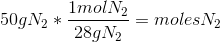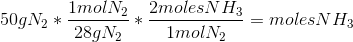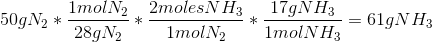### Example Question #2 : Stoichiometry

What is the percent composition by mass of oxygen in a molecule of glucose?

Possible Answers: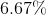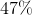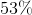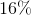Correct answer:Explanation:

The formula for glucose is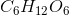.

First, we must find the molecular weight of the entire molecule of glucose. We do this individually for each element. Using oxygen as an example, we multiply the atomic mass (16) by the number of atoms per glucose molecule: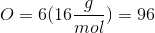We do similar calculations for carbon and hydrogen to find our total molecular weight for glucose.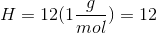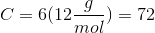Sum these values to find the molecular weight.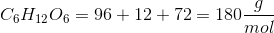Next, to find percent composition by mass for oxygen, we are being asked to find how much of one molecule of glucose's weight comes from oxygen. To do this we divide the mass of oxygen by the molecular weight: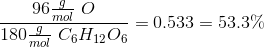Thus 53.3% of the mass of glucose comes from oxygen alone.

### Example Question #3 : Stoichiometry

What is the mass of 25 iron atoms?

Possible Answers: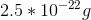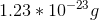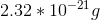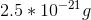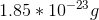Correct answer:Explanation:

First, convert the number of atoms to moles using Avogadro's number: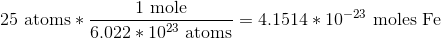Next, convert moles of iron to grams of iron using the molar mass: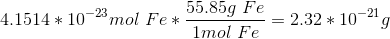### Example Question #4 : Stoichiometry

How many moles are in a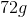sample of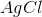?

Possible Answers: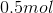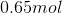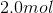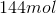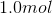Correct answer:Explanation:

To find the number of moles in a sample, use the periodic table to find the molar mass. Since the atomic weights of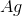and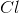are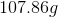and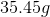, respectively, add the two atomic masses to determine the molar mass of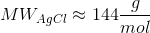Next, use dimensional analysis to find the number of moles in the given mass of the sample.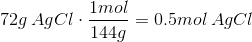### Example Question #5 : Stoichiometry

How many grams of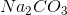are in 5.61mol of?

Possible Answers: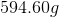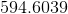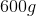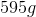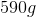Correct answer:Explanation:

Use the periodic table to compute the molecular mass of.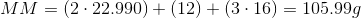Next, use dimensional analysis to convert grams to moles.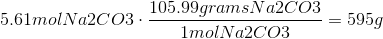Use the correct number of significant figures.

### Example Question #1 : Stoichiometry

A silicon microchip weighs 7.2mg. How many atoms of silicon are in the microchip?

Possible Answers: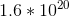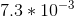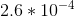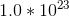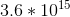Correct answer:Explanation:

First convert the 7.2mg of silicon into grams: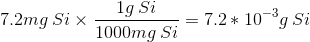Then convert grams of silicon into moles of silicon: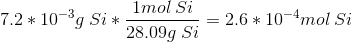Finally, convert moles of silicon into atoms using Avogadro's number: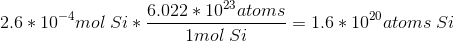Since 7.2mg of silicon is much less than 1 mole (which we know weighs 28.09g) our answer makes sense as it is much less than Avogadro's number.

### Example Question #7 : Stoichiometry

Blood contains various dissolved ions including calcium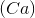, which is involved in many biological processes (like muscle contraction). One liter of blood from an unknown animal was shown to contain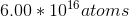of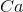. Calculate the mass of calcium atoms in one liter of blood.

Possible Answers: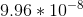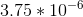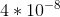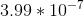None of these

Correct answer:

None of these

Explanation:

The answer is not present, butequates to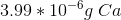.

Use Avogadro's number to convert from atoms to moles: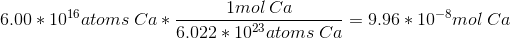Convert from moles ofto grams of: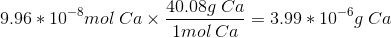### Example Question #8 : Stoichiometry

What is the atomic number of calcium?

Possible Answers:Correct answer:Explanation:

The atomic number is equal to the number of protons in an atom and can be determined using the periodic table. The atomic number is located on the top left of the box containing the desired chemical symbol. The chemical symbol for calcium is Ca and the atomic number is 20.

### Example Question #9 : Stoichiometry

How many neutrons does calcium-42 have?

Possible Answers: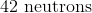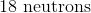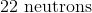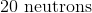Correct answer:Explanation:

The 42 in calcium-42 represents the mass number of calcium. The mass number equals the sum of the number of protons and the number of neutrons. Using the periodic table, the number of protons in calcium can be determined using the atomic number. The atomic number of calcium is 20.

Using all this information the number of neutrons can be determined by subtracting the number of protons from the atomic mass number. The number of neutrons is 22.

Atomic Mass Number = Protons + Neutrons

Atomic Mass Number - Protons = Neutrons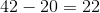### Example Question #10 : Stoichiometry

An atom contains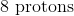and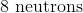. What is the mass number of this atom?

Possible Answers:Correct answer:Explanation:

The mass number is equal to the number of protons plus the number of neutrons in an atom.

Protons + Neutrons = Mass Number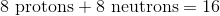← Previous 1 3

### All High School Chemistry Resources